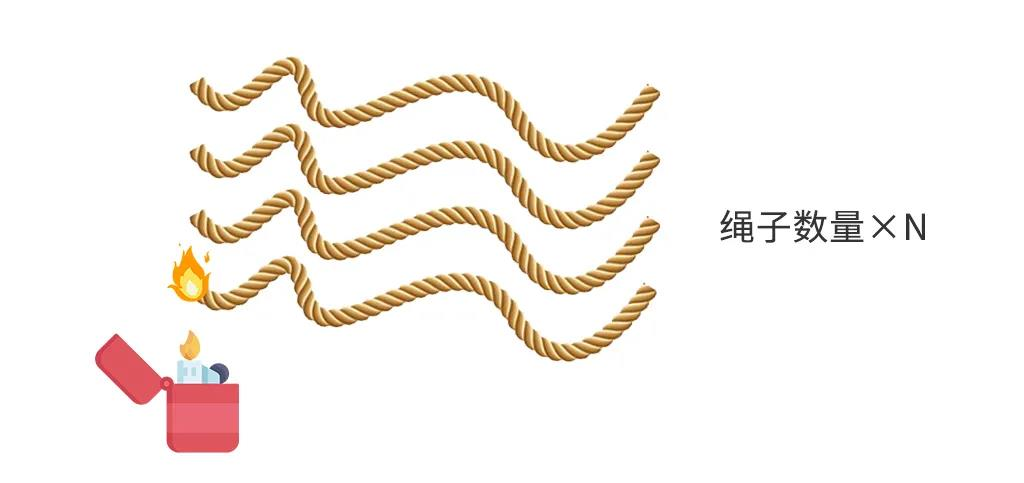# 学编程必看：10道逻辑思维测试题（附答案）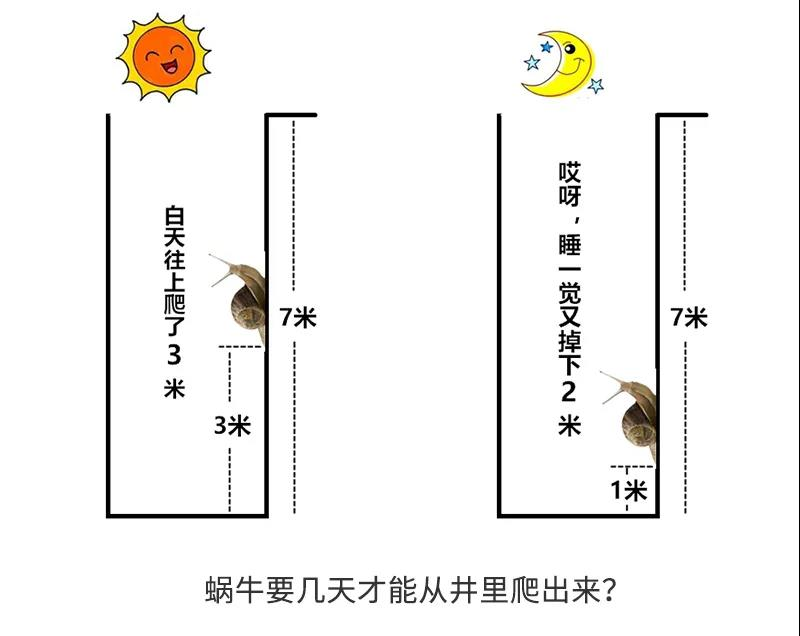（3-2）*（X-1）+3=7，解出方程式可得X=5。

3-2为每天蜗牛的实际上升高度，第X天白天蜗牛爬升3米即可爬出井口，那么X-1表示在第X天之前的累计爬升高度。1块钱买10个桃，剩10个核；

9个核换3个桃，加上余下的一个，剩4个核；

3个核换一个桃，加上余下的一个，剩2个核；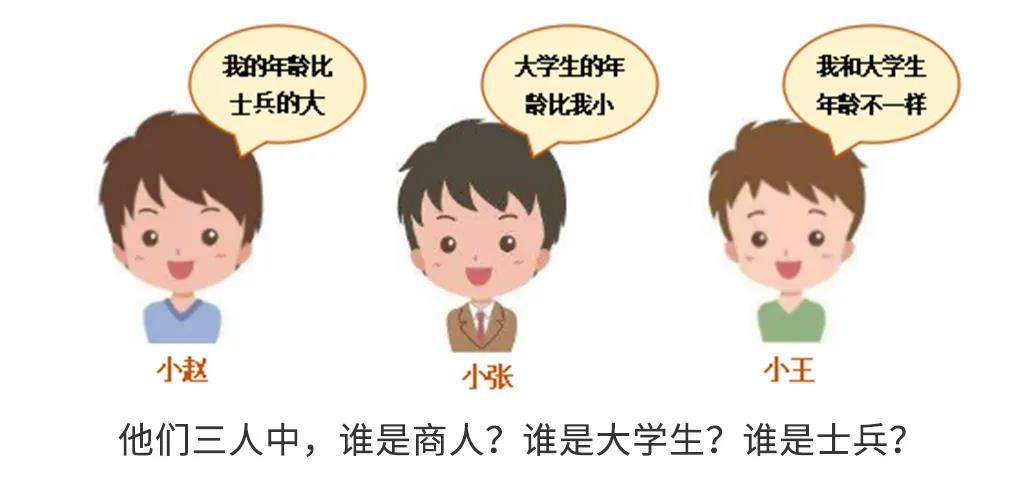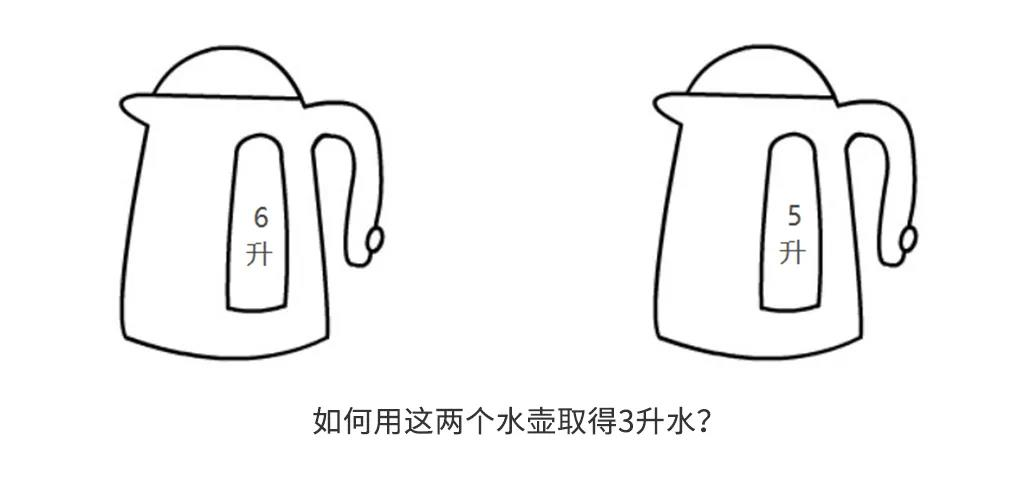6升水壶a，5升水壶b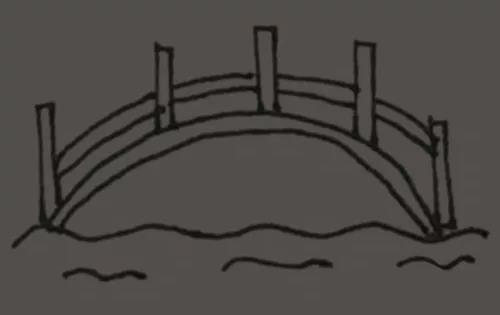2+1先过，用了2分钟；

5+10再过，用了10分钟；

2回来送手电筒，用了2分钟；

2+1过去，用了2分钟；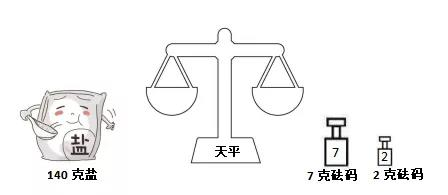①用天平将盐分成70g两份，即140 ->70 + 70

②用天平将其中一份70g的盐分成35g两份，即70 ->35 + 35

③把两个砝码放在天平两边，将35g的盐分成20克和15克，即35 + 2 + 7 ->(20 + 2) + (15 + 7)

90 = 20 + 70

50 = 15 + 35

①140 + 2 ->71 + (69 + 2)

②71 + 9 ->40 + (31 + 9)

③31 + 69 -> 50 + 50

90 = 50 + 40

①140 ->70 + 70

②70 ->61 + 9  将两个砝码放在一边，即可称出9g

③61 -> (9 +2) + 50

90 = 9 + 11 + 70

①140 ->70 + 70

②70 ->35 + 35

③35 + 70 + 2 + 7 ->(50 + 7) + (55 + 2)

90 = 55 + 35

①2 + 7 = 9

②2 + 7 + 9 =18

③7 + 18 = 23 + 2

50 = 23 + 9 +18

①140 + 2 ->71 + (69 + 2)

②7 = 2 + 5；69剩64

③2 + 5 + 7 = 14；64剩50

90 = 14 + 5 + 71

①2 + 7 = 9

②7 + 9 = 16

③9 + 16 = 25

50 = 25 + 16 + 9

①2 + 7 = 9

②7 + 9 = 2+ 14

③2 + 14 + 9 = 18 + 7

50 = 2 + 7 + 9 + 14 + 18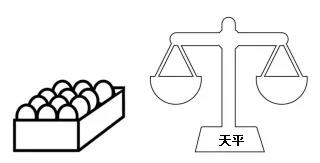▼ 第一种可能：（1,2,3,4）=（5,6,7,8）【第一次称重】

1）如果（6,7,8）=（9,10,11），说明坏鸡蛋是12。在这种情况下，只需要称2次就能找出坏鸡蛋。

2）如果（6,7,8）>（9,10,11），说明坏鸡蛋在（9,10,11）中，同时可以说明坏鸡蛋一定比好鸡蛋轻。接着称9和10。如果9=10，则说明11为坏鸡蛋；否则，轻的为坏鸡蛋。【第三次称重】

3）如果（6,7,8）<（9,10,11），与2）使用相同的方法称3次就可以得到坏鸡蛋。【第三次称重】

▼ 第二种可能：（1,2,3,4）≠（5,6,7,8）【第一次称重】

1）如果（1,2,5）=（3,4,6），说明坏鸡蛋一定在（7,8）中，而且坏鸡蛋一定比好鸡蛋轻。

2）如果（1,2,5）>（3,4,6），坏鸡蛋一定在（1,2,3,4,5,6）中，再继续称（2,3,5）和（1,4,7）。【第三次称重】

① 如果（2,3,5）=（1,4,7），说明6是坏鸡蛋。

② 如果（2,3,5）>（1,4,7），

③ 如果（2,3,5）

3）如果（1,2,5）